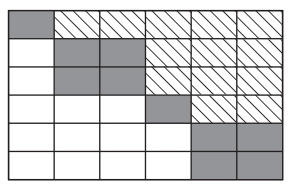# ordschur

Reorder eigenvalues in Schur factorization

## Syntax

``[US,TS] = ordschur(U,T,select)``
``[US,TS] = ordschur(U,T,keyword)``
``[US,TS] = ordschur(U,T,clusters)``

## Description

````[US,TS] = ordschur(U,T,select)` reorders the Schur factorization `X = U*T*U'` produced by `[U,T] = schur(X)` and returns the reordered Schur matrix `TS` and the orthogonal matrix `US`, such that `X = US*TS*US'`.In this reordering, the selected cluster of eigenvalues appears in the leading (upper left) diagonal blocks of the quasitriangular Schur matrix `TS`. The leading columns of `US` span the corresponding invariant subspace. The logical vector `select` specifies the selected cluster as `e(select)`, where `e = ordeig(T)`.```
````[US,TS] = ordschur(U,T,keyword)` sets the selected cluster to include all eigenvalues in the region specified by `keyword`.```

example

````[US,TS] = ordschur(U,T,clusters)` reorders multiple clusters simultaneously. `ordschur` sorts the specified clusters in descending order along the diagonal of `TS`, with the cluster of highest index appearing in the upper left corner.```

## Examples

collapse all

Compute the Schur factors of a matrix, then reorder the factors according to a specified ordering of the eigenvalues.

Find the Schur factorization of a matrix `X`. The Schur factorization produces an upper quasitriangular matrix `T` and a unitary matrix `U` such that $\mathit{X}={\mathrm{UTU}}^{*}$.

```X = magic(6); [U,T] = schur(X)```
```U = 6×6 0.4082 -0.2887 0.4082 0.5749 0.5000 -0.0530 0.4082 0.5774 0.4082 -0.3333 0.0000 -0.4714 0.4082 -0.2887 0.4082 -0.2416 -0.5000 0.5244 0.4082 0.2887 -0.4082 0.5749 -0.5000 -0.0530 0.4082 -0.5774 -0.4082 -0.3333 -0.0000 -0.4714 0.4082 0.2887 -0.4082 -0.2416 0.5000 0.5244 ```
```T = 6×6 111.0000 0.0000 0.0000 0.0000 0.0000 -0.0000 0 27.0000 0.0000 -28.3164 -15.5885 -13.0454 0 0 -27.0000 18.0000 22.0454 -12.7279 0 0 0 9.7980 0.0000 6.9282 0 0 0 0 -0.0000 0.0000 0 0 0 0 0 -9.7980 ```

Since `T` is triangular, the diagonal of `T` contains the eigenvalues of the original matrix `X`.

Reorder the Schur factorization so that the eigenvalues are in two clusters, with the cluster of negative eigenvalues appearing first along the diagonal of `TS`.

`[US,TS] = ordschur(U,T,'lhp')`
```US = 6×6 -0.4082 0.2887 -0.2746 -0.4082 -0.4826 0.5244 -0.4082 0.2887 -0.2990 -0.4082 0.5213 -0.4714 -0.4082 -0.5774 0.5736 -0.4082 -0.0386 -0.0530 0.4082 -0.2887 -0.2075 -0.4082 0.5151 0.5244 0.4082 -0.2887 -0.3662 -0.4082 -0.4765 -0.4714 0.4082 0.5774 0.5736 -0.4082 -0.0386 -0.0530 ```
```TS = 6×6 -27.0000 -19.0919 18.6997 0.0000 9.7888 -12.7279 0 -0.0000 -0.3800 0.0000 15.6493 -15.5227 0 0 -9.7980 -0.0000 2.4773 -8.7185 0 0 0 111.0000 -0.0000 -0.0000 0 0 0 0 27.0000 -26.3600 0 0 0 0 0 9.7980 ```

## Input Arguments

collapse all

Unitary matrix, specified as the matrix `U` returned by `[U,T] = schur(X)`. The matrix `U` satisfies the properties `X = U*T*U'` and ```U'*U = eye(size(X))```.

If `U` and `T` do not form a valid Schur decomposition, then `ordschur` does not produce an error and returns incorrect results.

Data Types: `single` | `double`
Complex Number Support: Yes

Schur matrix, specified as the upper quasitriangular matrix `T` returned by `[U,T] = schur(X)`. The matrix `T` satisfies `X = U*T*U'`.

If `U` and `T` do not form a valid Schur decomposition, then `ordschur` does not produce an error and returns incorrect results.

Data Types: `single` | `double`
Complex Number Support: Yes

Cluster selector, specified as a logical vector with length equal to the number of eigenvalues. The eigenvalues appear along the diagonal of the matrix `T` produced by ```[U,T] = schur(X)```.

Data Types: `logical`

Eigenvalue region keyword, specified as one of the options in this table.

Option

Selected Region

(`e = ordeig(T)`)

`'lhp'`

Left-half plane (```real(e) < 0```)

`'rhp'`

Right-half plane (```real(e) >= 0```)

`'udi'`

Interior of unit disk (```abs(e) < 1```)

`'udo'`

Exterior of unit disk (```abs(e) >= 1```)

Cluster indices, specified as a vector of positive integers with length equal to the number of eigenvalues. `clusters` assigns each eigenvalue returned by `e = ordeig(T)` to a different cluster. All eigenvalues with the same index value in `clusters` form one cluster.

Example: `ordschur(U,T,[1 1 2 3 3])` groups five eigenvalues into three clusters.

Data Types: `single` | `double`

## Output Arguments

collapse all

Reordered matrices, returned as matrices that satisfy ```X = US*TS*US'```.

`US` is a unitary matrix, and `TS` is quasitriangular.

collapse all

### Quasitriangular

An upper quasitriangular matrix can result from the Schur decomposition or generalized Schur (QZ) decomposition of real matrices. These matrices are block upper triangular, with 1-by-1 and 2-by-2 blocks along the diagonal.The eigenvalues of these diagonal blocks are also the eigenvalues of the matrix. The 1-by-1 blocks correspond to real eigenvalues, and the 2-by-2 blocks correspond to complex conjugate eigenvalue pairs.

## Tips

• If `T` has complex conjugate pairs (nonzero elements on the subdiagonal), then you should move the pair to the same cluster. Otherwise, `ordschur` acts to keep the pair together:

• If `select` is not the same for two eigenvalues in a conjugate pair, then `ordschur` treats both as selected.

• If `clusters` is not the same for two eigenvalues in a conjugate pair, then `ordschur` treats both as part of the cluster with larger index.

 Kressner, Daniel. “Block Algorithms for Reordering Standard and Generalized Schur Forms.” ACM Transactions on Mathematical Software 32, no. 4 (December 2006): 521–532. https://doi.org/10.1145/1186785.1186787.